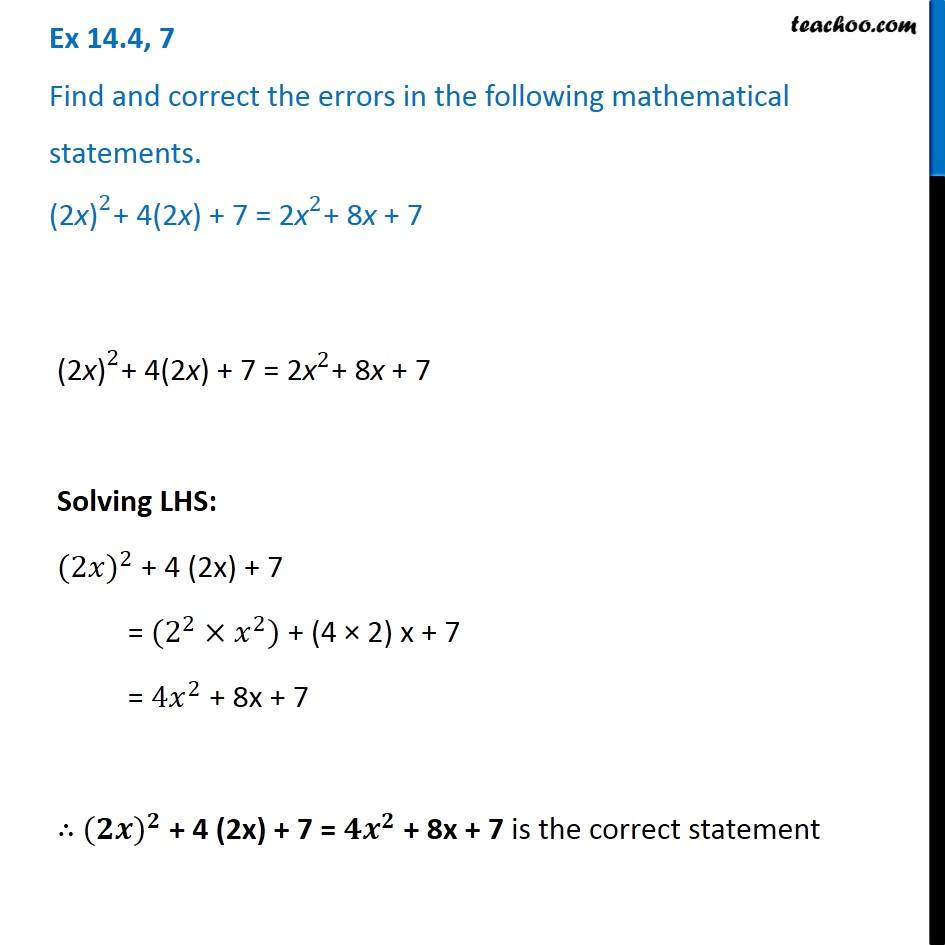1. Chapter 14 Class 8 Factorisation
2. Concept wise
3. Finding Errors

Transcript

Ex 14.4, 7 Find and correct the errors in the following mathematical statements. 〖"(2x)" 〗^2+ 4(2x) + 7 = 〖"2x" 〗^2+ 8x + 7 〖"(2x)" 〗^2+ 4(2x) + 7 = 〖"2x" 〗^2+ 8x + 7 Solving LHS: 〖(2𝑥)〗^2 + 4 (2x) + 7 = (2^2×𝑥^2) + (4 × 2) x + 7 = 〖4𝑥〗^2 + 8x + 7 ∴ 〖(𝟐𝒙)〗^𝟐 + 4 (2x) + 7 = 〖𝟒𝒙〗^𝟐 + 8x + 7 is the correct statement